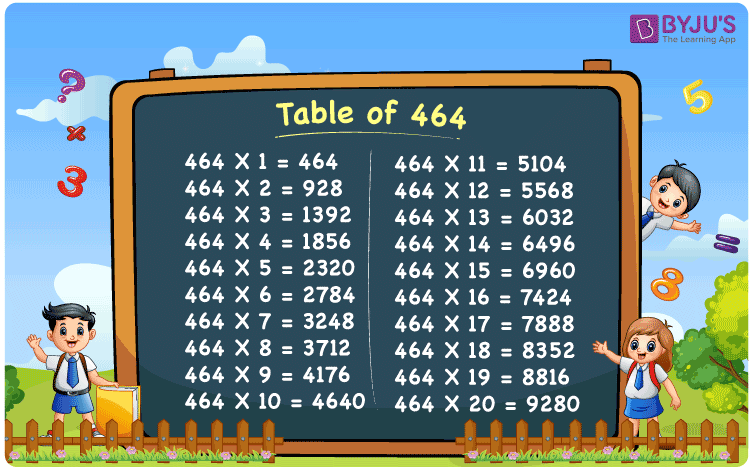Checkout JEE MAINS 2022 Question Paper Analysis : Checkout JEE MAINS 2022 Question Paper Analysis :

# Table of 464

The Multiplication Table for 464 is nothing more than the repetitive addition of the number 464. 464 is an even number that is divisible by 2. Note that 464 is also divisible by 4. Observe that all the multiples of 464 are also divisible by 4. You can read more on Divisibility rules.

## Table of 464 Chart## What is the 464 Times Table?

In the table of 464, we are repeatedly adding 464 multiple times. And the table below gives a repetitive addition 10 times for the number 464.

 464 × 1 = 464 464 464 × 2 = 928 464 + 464 = 928 464 × 3 = 1392 464 + 464 + 464 = 1392 464 × 4 = 1856 464 + 464 + 464 + 464 = 1856 464 × 5 = 2320 464 + 464 + 464 + 464 + 464 = 2320 464 × 6 = 2784 464 + 464 + 464 + 464 + 464 + 464 = 2784 464 × 7 = 3248 464 + 464 + 464 + 464 + 464 + 464 + 464 = 3248 464 × 8 = 3712 464 + 464 + 464 + 464 + 464 + 464 + 464 + 464 = 3712 464 × 9 = 4176 464 + 464 + 464 + 464 + 464 + 464 + 464 + 464 + 464 = 4176 464 × 10 = 4640 464 + 464 + 464 + 464 + 464 + 464 + 464 + 464 + 464 + 464 = 4640

## Multiplication Table of 464

Given below is the multiplication table of 464 being done 20 times. The below table helps you with multiplication problems that require the tables of 464.

 464 × 1 = 464 464 × 2 = 928 464 × 3 = 1392 464 × 4 = 1856 464 × 5 = 2320 464 × 6 = 2784 464 × 7 = 3248 464 × 8 = 3712 464 × 9 = 4176 464 × 10 = 4640 464 × 11 = 5104 464 × 12 = 5568 464 × 13 = 6032 464 × 14 = 6496 464 × 15 = 6960 464 × 16 = 7424 464 × 17 = 7888 464 × 18 = 8352 464 × 19 = 8816 464 × 20 = 9280

## Solved Example on Table of 464

Example:

Neena has a Rs 2000 note. She spent Rs 1392 on 3 dresses, each costing the same price. How much does each dress cost?

Solution: Rs 464.

1392 on 3 dresses. So each dress is 1392 / 3 = 464.

## Frequently Asked Questions on Table of 464

### Question 1: Is 464 a perfect square number?

Answer: 464 is not a perfect square number. 484 is a perfect square number.

### Question 2: Is 464 a multiple of 64?

Answer: No. 464 is not a multiple of 64.

### Question 3: What are the factors of 464?

Answer: The factors of 464 are 1, 2, 4, 8, 16, 29, 58, 116, 232, and 464.